# NCERT Solutions For Class 10 Maths Chapter 2 Exercise 2.2 – Polynomials

Download NCERT Solutions For Class 10 Maths Chapter 2 Exercise 2.2 – Polynomials. This Exercise contains 2 questions, for which detailed answers have been provided in this note. In case you are looking at studying the remaining Exercise for Class 10 for Maths NCERT solutions for other Chapters, you can click the link at the end of this Note.### NCERT Solutions For Class 10 Maths Chapter 2 Exercise 2.2 – Polynomials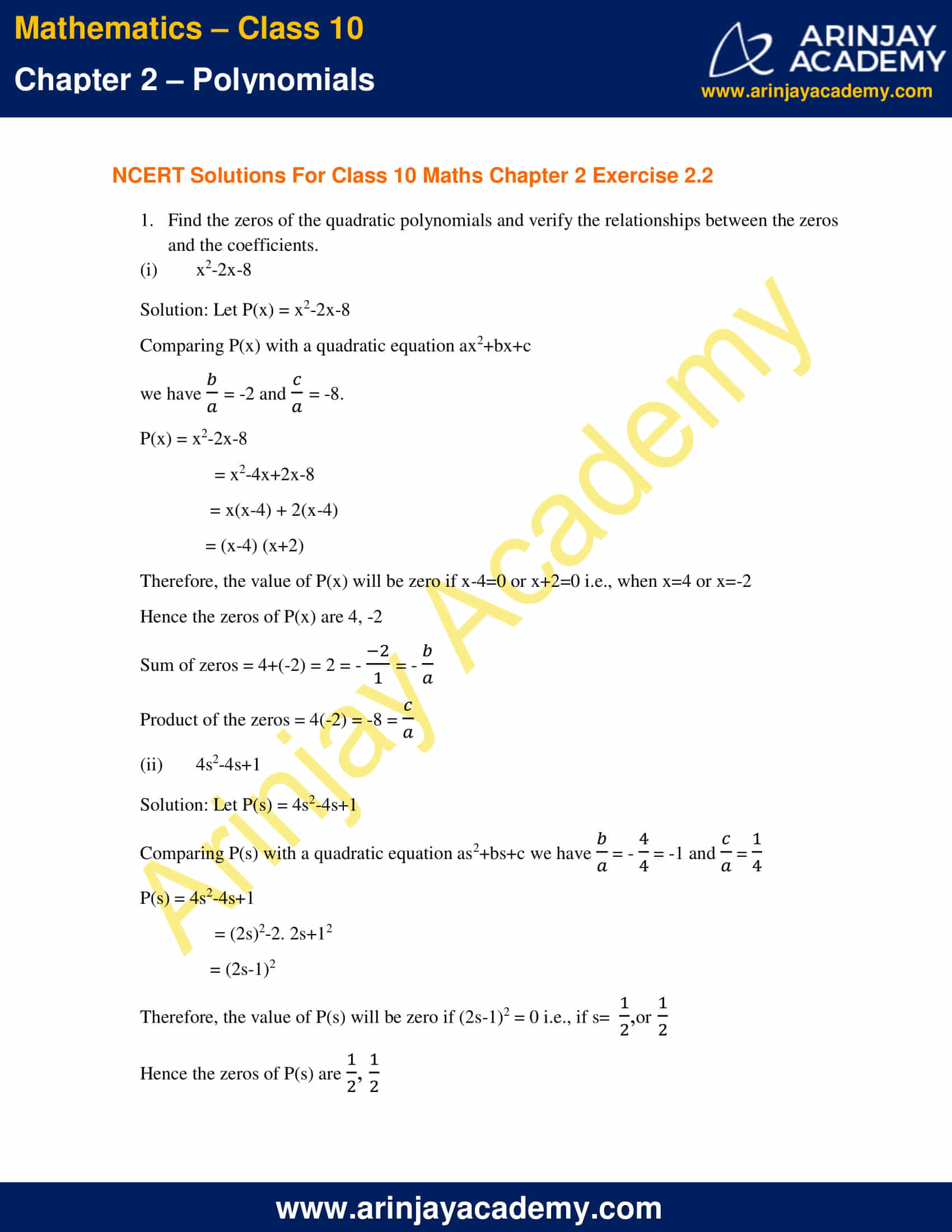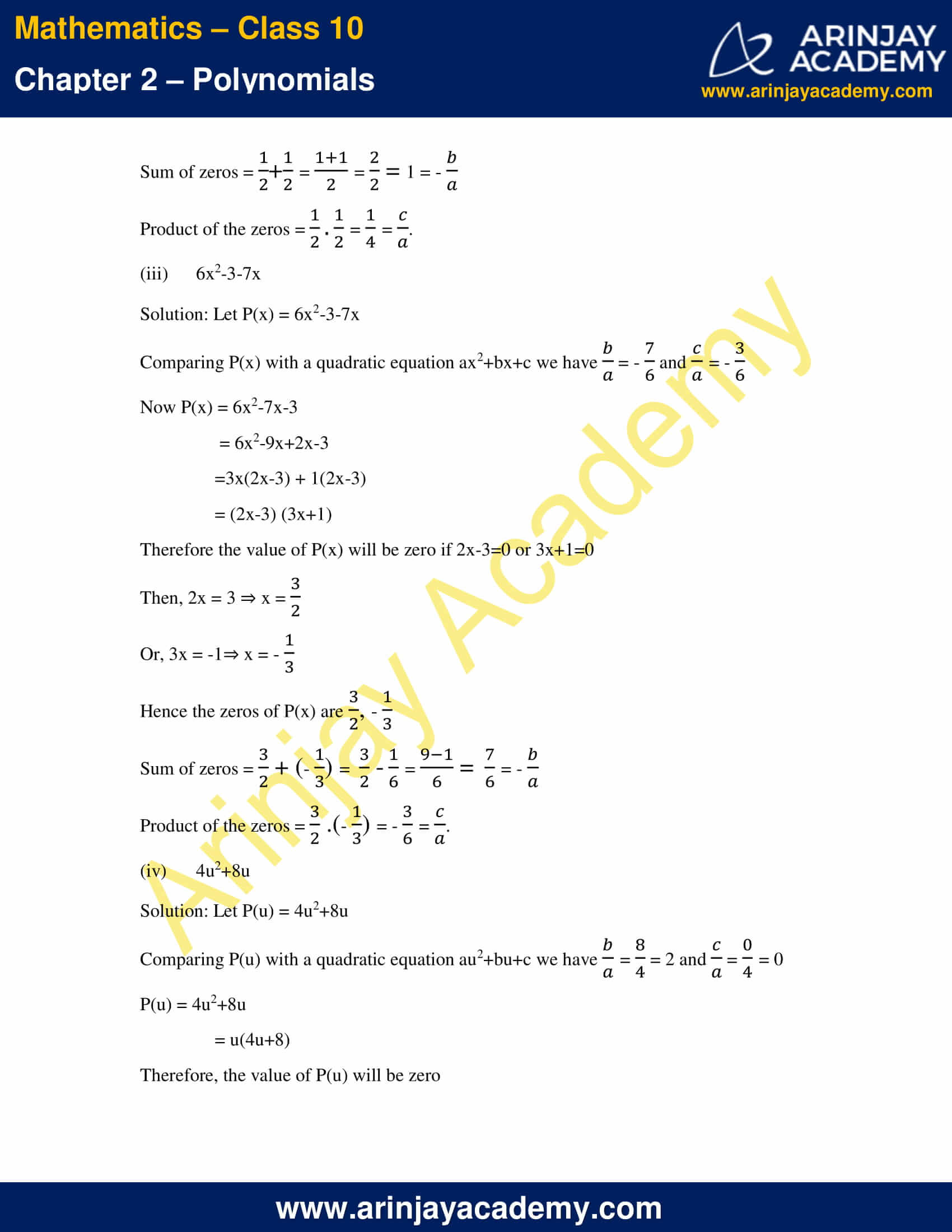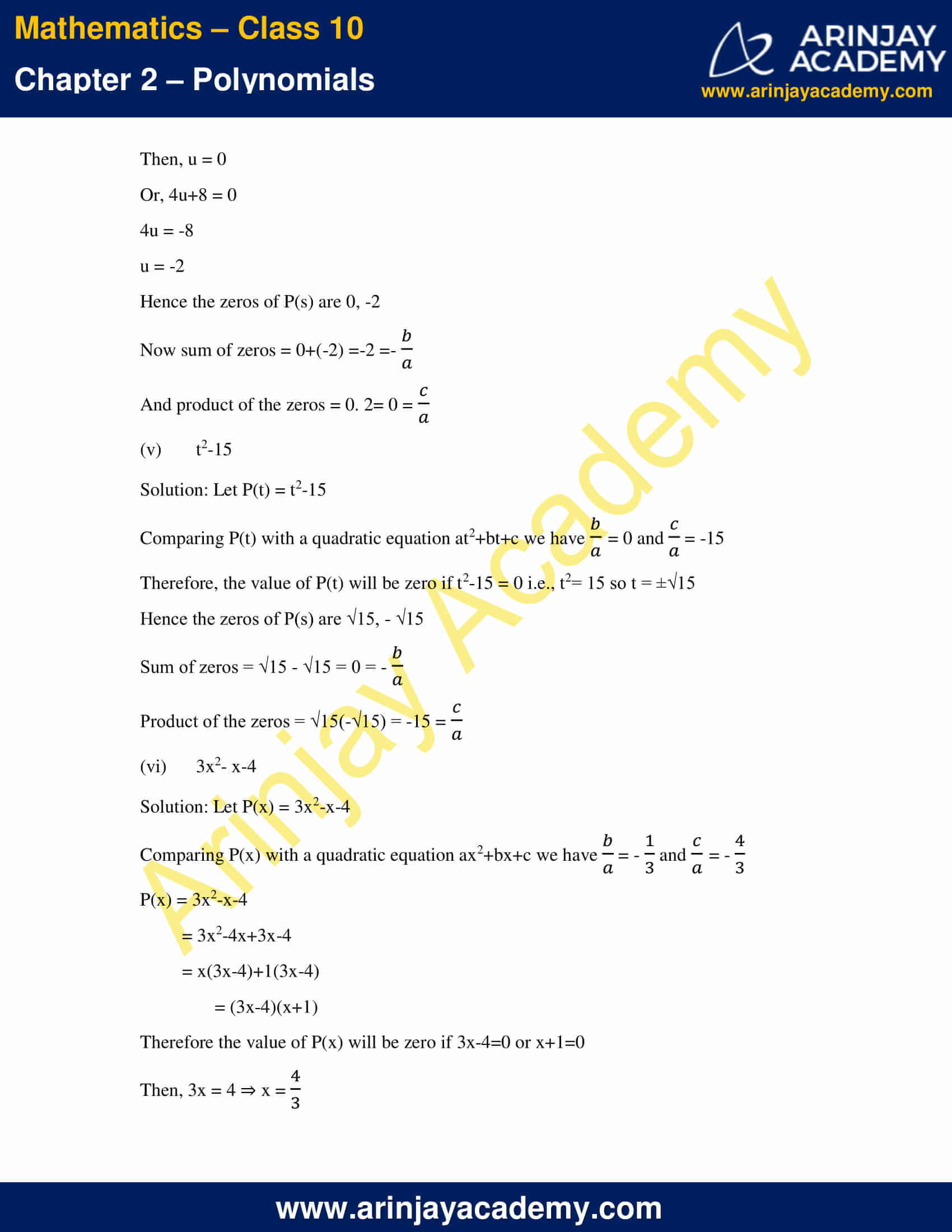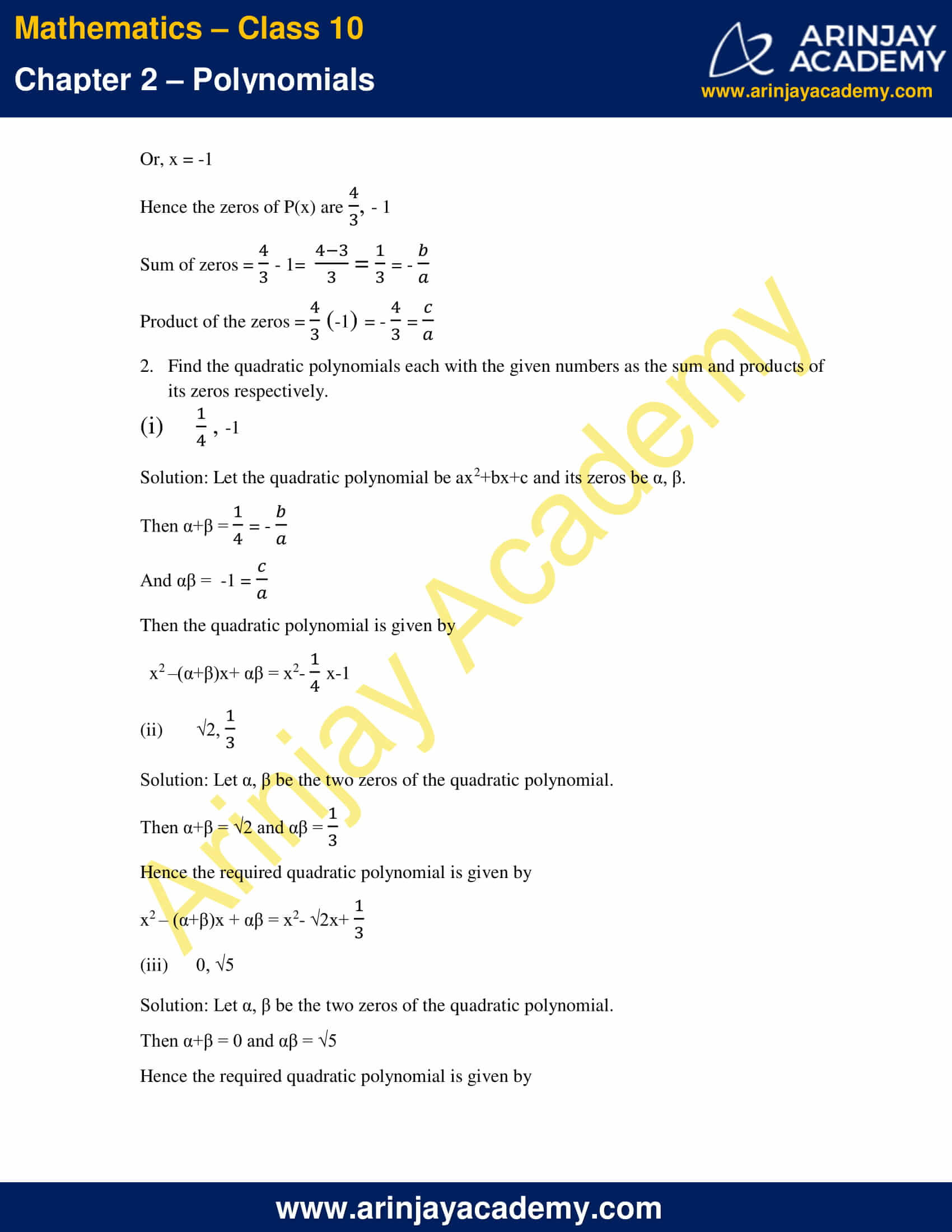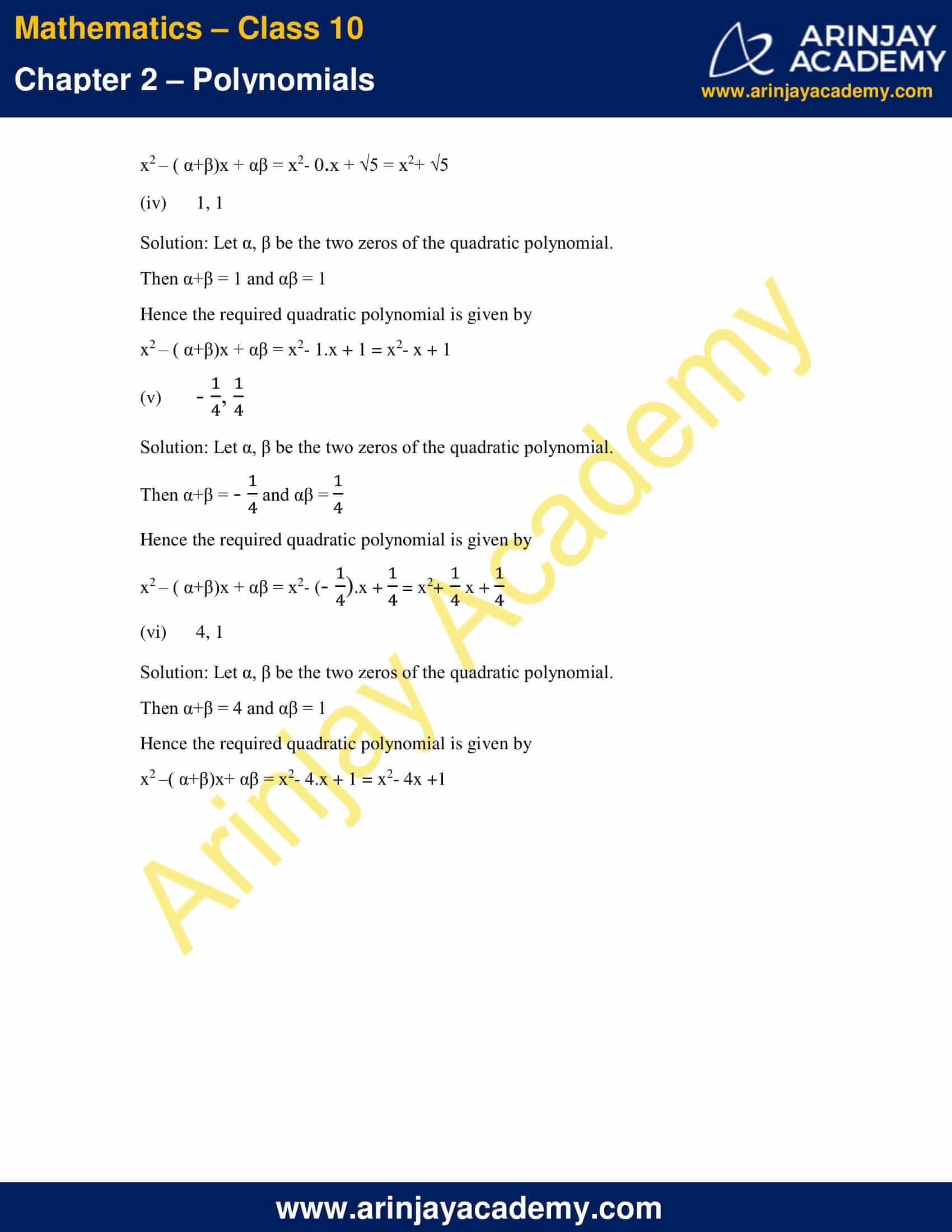NCERT Solutions For Class 10 Maths Chapter 2 Exercise 2.2 – Polynomials

1. Find the zeros of the quadratic polynomials and verify the relationships between the zeros and the coefficients.

(i) x2-2x-8

Solution:

Let, P(x) = x2-2x-8

Comparing P(x) with a quadratic equation ax2+bx+c

we have b/a = -2 and c/a = -8.

P(x) = x2-2x-8

= x2-4x+2x-8

= x(x-4) + 2(x-4)

= (x-4) (x+2)

Therefore, the value of P(x) will be zero if x-4=0 or x+2=0 i.e., when x=4 or x=-2

Hence the zeros of P(x) are 4, -2

Sum of zeros = 4+(-2) = 2 = -(-2/1) = -(b/a)

Product of the zeros = 4(-2) = -8 = c/a

(ii) 4s2-4s+1

Solution:

Let P(s) = 4s2-4s+1

Comparing P(s) with a quadratic equation as2+bs+c we have b/a = -(4/4)  = -1 and (c/a) = 1/4

P(s) = 4s2-4s+1

= (2s)2-2. 2s+12

= (2s-1)2

Therefore, the value of P(s) will be zero if (2s-1)2 = 0 i.e., if s = 1/2 ,or 1/2

Hence the zeros of P(s) are 1/2, 1/2

Sum of zeros = 1/2 + 1/2 = (1+1)/2 = 2/2 = 1 = -b/a

Product of the zeros = (1/2).(1/2) = 1/4 = c/a

(iii) 6x2-3-7x

Solution:

Let, P(x) = 6x2-3-7x

Comparing P(x) with a quadratic equation ax2+bx+c we have b/a = -7/6  and c/a = – 3/6

Now P(x) = 6x2-7x-3

= 6x2-9x+2x-3

=3x(2x-3) + 1(2x-3)

= (2x-3) (3x+1)

Therefore the value of P(x) will be zero if 2x-3=0 or 3x+1=0

Then, 2x = 3

x = 3/2

Or, 3x = -1

x = -(1/3)

Hence the zeros of P(x) are 3/2, -(1/3)

Sum of zeros = 3/2 + (-1/3) = 3/2  – 1/6 = (9-1)/6 = 7/6  = -(b/a)

Product of the zeros = (3/2).(-1/3) = -(3/6)  = c/a

(iv) 4u2+8u

Solution:

Let, P(u) = 4u2+8u

Comparing P(u) with a quadratic equation au2+bu+c we have b/a = 8/4 = 2 and c/a = 0/4 = 0

P(u) = 4u2+8u

= u(4u+8)

Therefore, the value of P(u) will be zero

Then, u = 0

Or, 4u+8 = 0

4u = -8

u = -2

Hence the zeros of P(s) are 0, -2

Now sum of zeros = 0+(-2) =-2 =-(b/a)

And product of the zeros = 0. 2= 0 = c/a

(v) t2-15

Solution:

Let P(t) = t2-15

Comparing P(t) with a quadratic equation at2+bt+c we have b/a = 0 and c/a = -15

Therefore, the value of P(t) will be zero if t2-15 = 0 i.e., t2= 15 so t = ±√15

Hence the zeros of P(s) are √15, – √15

Sum of zeros = √15 – √15 = 0 = -(b/a)

Product of the zeros = √15(-√15) = -15 = c/a

(vi) 3x2– x-4

Solution:

Let P(x) = 3x2-x-4

Comparing P(x) with a quadratic equation ax2+bx+c we have b/a = -(1/3) and (c/a) = -(4/3)

P(x) = 3x2-x-4

= 3x2-4x+3x-4

= x(3x-4)+1(3x-4)

= (3x-4)(x+1)

Therefore the value of P(x) will be zero if 3x-4=0 or x+1=0

Then, 3x = 4

x = 4/3

Or, x = -1

Hence the zeros of P(x) are 4/3, – 1

Sum of zeros = (4/3) – 1= (4-3)/3  = 1/3 = -(b/a)

Product of the zeros = (4/3).(-1) = -(4/3)  = c/a

2. Find the quadratic polynomials each with the given numbers as the sum and products of its zeros respectively.

(i) (1/4), -1

Solution: Let the quadratic polynomial be ax2+bx+c and its zeros be α, β.

Then α+β = (1/4) = -(b/a)

And αβ =  -1 = (c/a)

Then the quadratic polynomial is given by

x2 –(α+β)x+ αβ = x2– (1/4)x – 1

(ii) √2, 1/3

Solution: Let α, β be the two zeros of the quadratic polynomial.

Then α+β = √2 and αβ = 1/3

Hence the required quadratic polynomial is given by

x2 – (α+β)x + αβ = x2– √2x + (1/3)

(iii) 0, √5

Solution: Let α, β be the two zeros of the quadratic polynomial.

Then α+β = 0 and αβ = √5

Hence the required quadratic polynomial is given by

x2 – ( α+β)x + αβ = x2– 0.x + √5 = x2+ √5

(iv) 1, 1

Solution: Let α, β be the two zeros of the quadratic polynomial.

Then α+β = 1 and αβ = 1

Hence the required quadratic polynomial is given by

x2 – ( α+β)x + αβ = x2– 1.x + 1 = x2– x + 1

(v) -(1/4) , (1/4)

Solution: Let α, β be the two zeros of the quadratic polynomial.

Then α+β = -(1/4)  and αβ = 1/4

Hence the required quadratic polynomial is given by

x2 – ( α+β)x + αβ = x2– (-1/4).x + (1/4) = x2+ (1/4)x + 1/4

(vi) 4, 1

Solution: Let α, β be the two zeros of the quadratic polynomial.

Then α+β = 4 and αβ = 1

Hence the required quadratic polynomial is given by

x2 –( α+β)x+ αβ = x2– 4.x + 1 = x2– 4x +1

NCERT Solutions for Class 10 Maths Chapter 2 Exercise 2.2 – Polynomials, has been designed by the NCERT to test the knowledge of the student on the topic – Relationship between Zeroes and Coefficients of a Polynomial